# Electrodynamics

## Equations / Theorems

### Guass' Law for electrical field of closed surface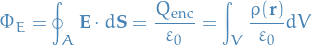for any closed surface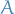and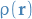is the surface charge-density.

### Poisson's Equation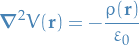where we've used Gauss' Law and the fact that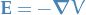.

#### Boundary conditions

We have the standard boundary conditions in Electrostatiscs: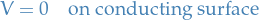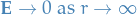Note that since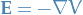we basically have boundary conditions for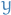and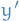in the "normal" ODE sense.

#### Properties

##### Uniqueness

For any two different solutions of the Poisson's equation, the solutions only differ by a constant.

#### Laplace's equation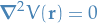which is a special case of the Poisson's Equation, where we have space absent of free charges .

#### Examples

The charge density within the follow region is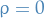, and thus we have the Laplace's equation with the boundary conditions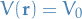where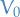, i.e. the potential at the boundary of the inner (non-charge) region is equal to the potential of the inner boundary of the conductor (since they're obviously the same boundary).

#### Method of Images

1. "Imagine" a charge s.t. we get a homogenous PDE (equiv. Laplace's equation)
2. Solve homogenous PDE / Laplacian using [BROKEN LINK: No match for fuzzy expression: *Laplace's%20equation%20in%20cylindrical%20coordinates], i.e. assume a solution of the form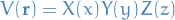3. Then find a special solution to the non-homogenous PDE
4. The linear combination of these two then form the general solution to the problem

There is one issue that one my bring up; is this solution unique? As it turns out, it is!

### Maxwell's equations

1. Gauss's law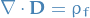2. Guass's law for magnetism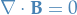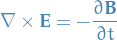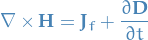•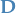is the displacement field, which accounts for the effect of free and bound charge within materials while its sources are the free charges only
•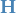is the magnetizing field
•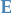is the electric field
•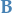is the magnetic field
•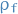is the free charge density